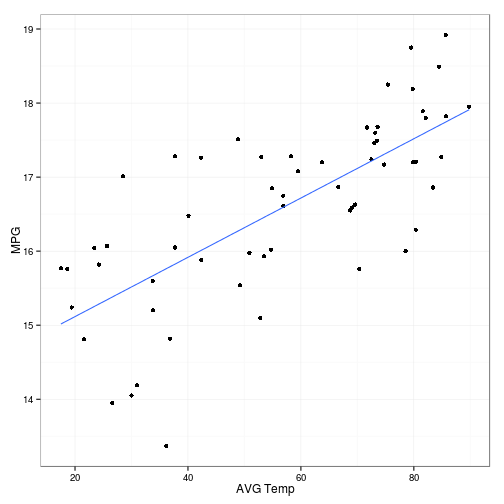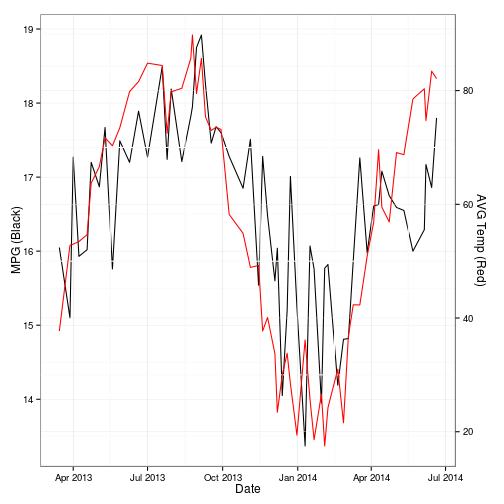## Monday, December 9, 2013

### Graphing my MPG vs Temperature

Updated: 7/9/2014

I use Fuelly to track the fuel mileage in my Toyota Tundra pickup truck. They have a mobile app, so while I'm waiting for the truck to fill up, I log the odometer, and the number of gallons that I put in and then also the price per gallon of fuel and an estimate of my city driving percentage.

Any how, I've noticed a substantial drop in fuel economy when the weather turns cold. So, remembering that I've decided to record the temperature at O'hare every day, I figured I'd try to take a look at the data. Now, I live 40 miles or so north of O'hare, so it's not perfect, but it was a good estimate.

To merge the daily temperature data with the sporadic fillup data, I chose to take the average high temperature for the days between the fillup[i] and fillup[i-1] to use as temperature[i]. I hope that makes sense. Basically, I take the average temperature over the tank of gas.

Let's see what a simple graph of average temperature by MPG looks like…

``````ggplot(fuel, aes(x=avg.temp, y=mpg)) + geom_point() +
geom_smooth(method = lm, se=FALSE) + xlab("AVG Temp") +
ylab("MPG") + theme_bw()
``````There's a fairly obvious pattern here. As temperature goes up, my MPG goes up, just as I suspected was happening.

Now, I know this next graph goes against normal data visualization guidelines, but I just think it makes the relationship easier to understand for a lay person. Here, I've plotted both temperate and MPG on the same graph, with MPG in black with it's axis on the left and temperature in red with it's axis on the right.

``````grid.newpage()

# two plots
p1 <- ggplot(fuel, aes(x=date, y=mpg)) + geom_line() +
theme_bw() + xlab("Date") + ylab("MPG (Black)")
p2 <- ggplot(fuel, aes(x=date, y=avg.temp)) +
xlab("Date") + ylab("AVG Temp (Red)") +
geom_line(colour="red") + theme_bw() +
theme(axis.title.y=element_text(angle = -90)) %+replace%
theme(panel.background = element_rect(fill = NA))

# extract gtable
g1 <- ggplot_gtable(ggplot_build(p1))
g2 <- ggplot_gtable(ggplot_build(p2))

# overlap the panel of 2nd plot on that of 1st plot
pp <- c(subset(g1\$layout, name == "panel", se = t:r))
g2\$grobs[[which(g2\$layout\$name == "panel")]],
pp\$t, pp\$l, pp\$b, pp\$l)

# axis tweaks
alab <- g2\$grobs[[which(g2\$layout\$name=="ylab")]]
ia <- which(g2\$layout\$name == "axis-l")
ga <- g2\$grobs[[ia]]
ax <- ga\$children[]
ax\$widths <- rev(ax\$widths)
ax\$grobs <- rev(ax\$grobs)
ax\$grobs[]\$x <- ax\$grobs[]\$x - unit(1, "npc") +
unit(0.15, "cm")
length(g\$widths) - 1 )
length(g\$widths) - 1 )
g <- gtable_add_grob(g, ax, pp\$t, length(g\$widths) - 2, pp\$b)
g <- gtable_add_grob(g, alab, pp\$t, length(g\$widths) - 1, pp\$b)

grid.draw(g)
``````Credit to this guy, for his dual axis ggplot2 code.

1.